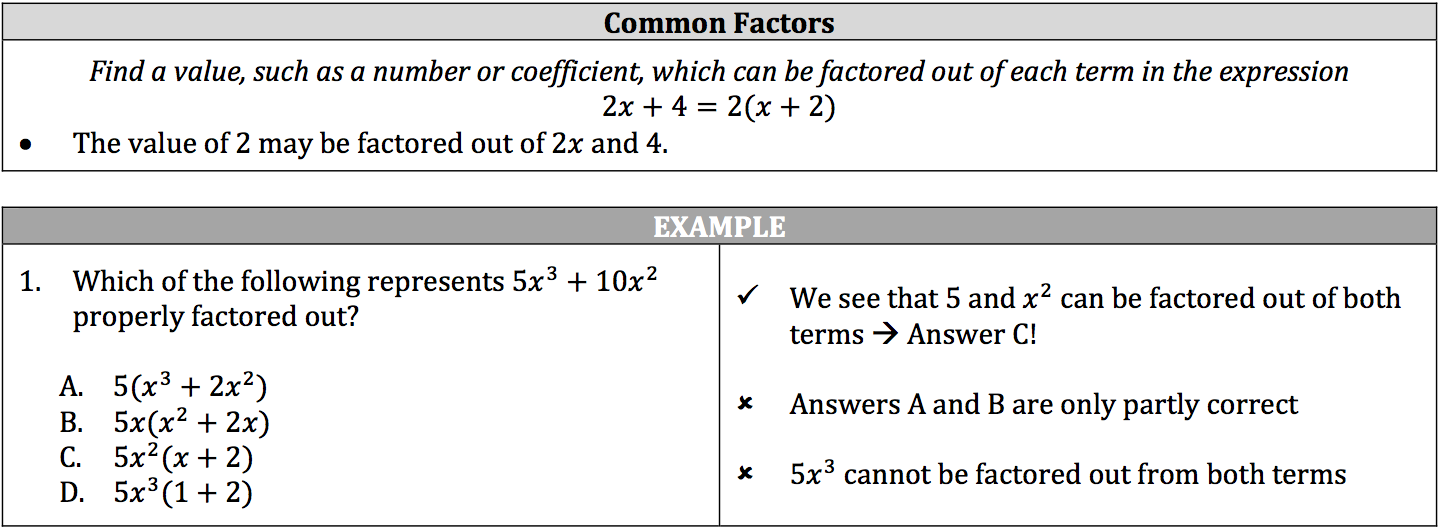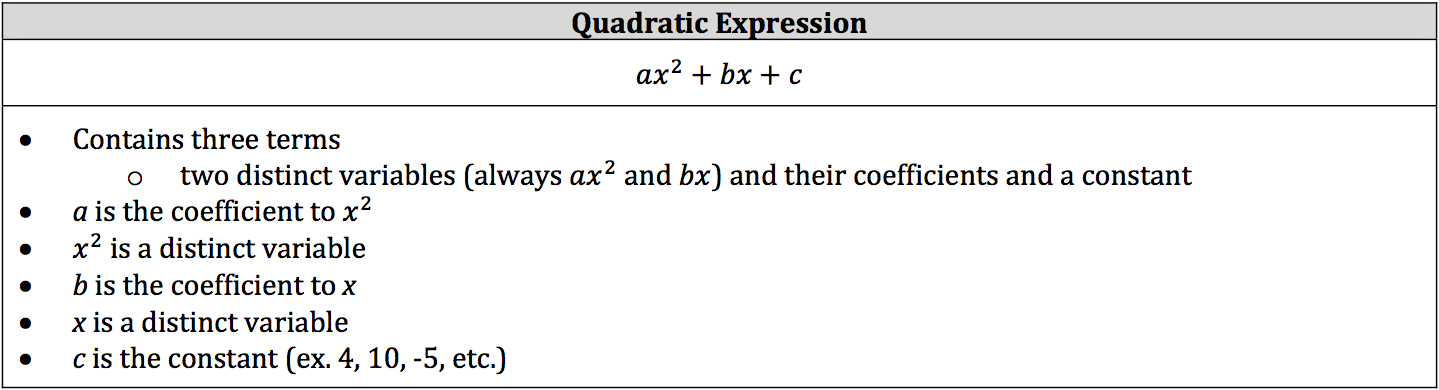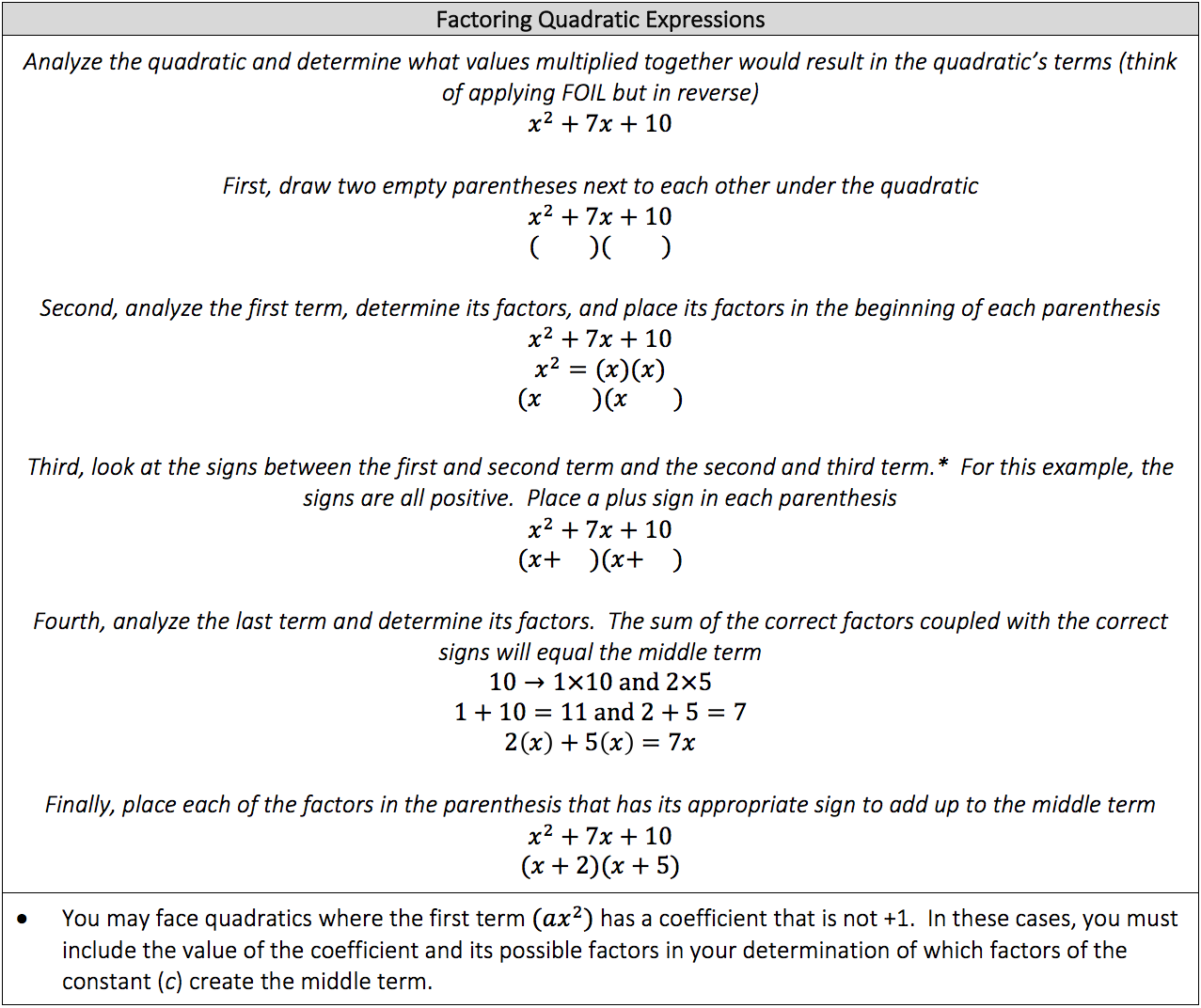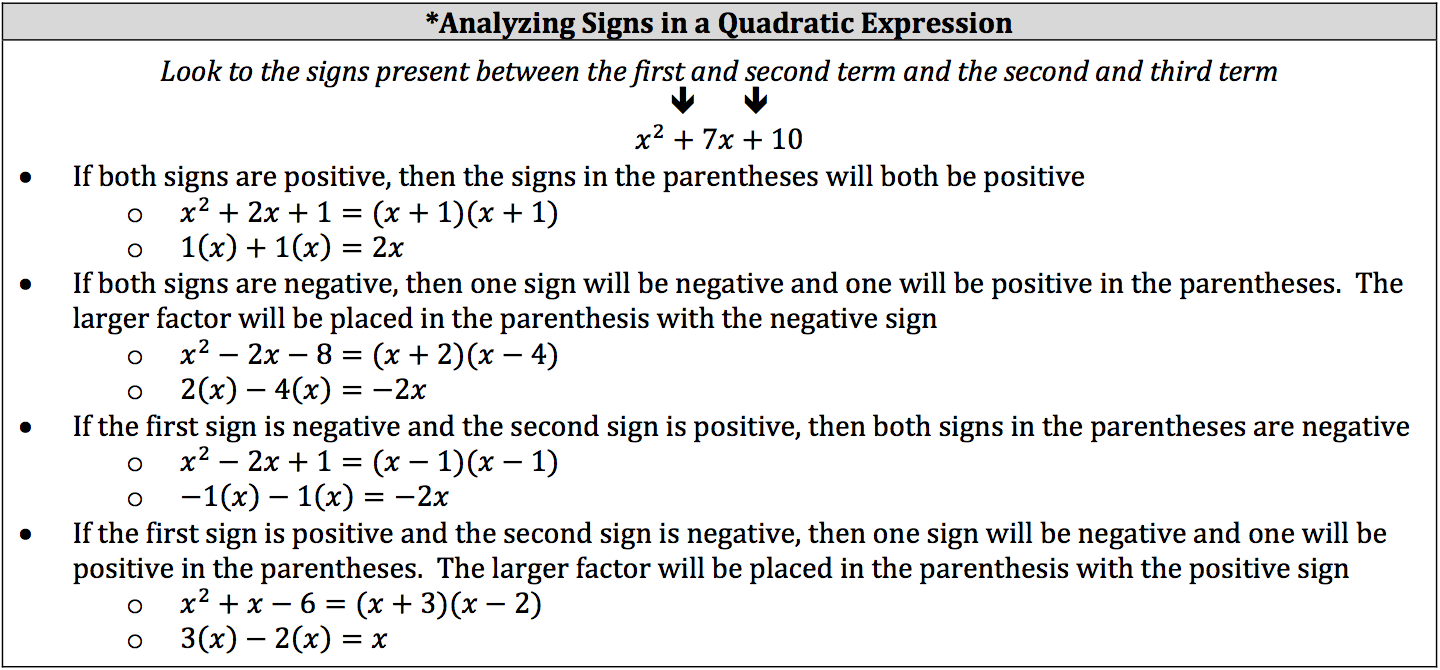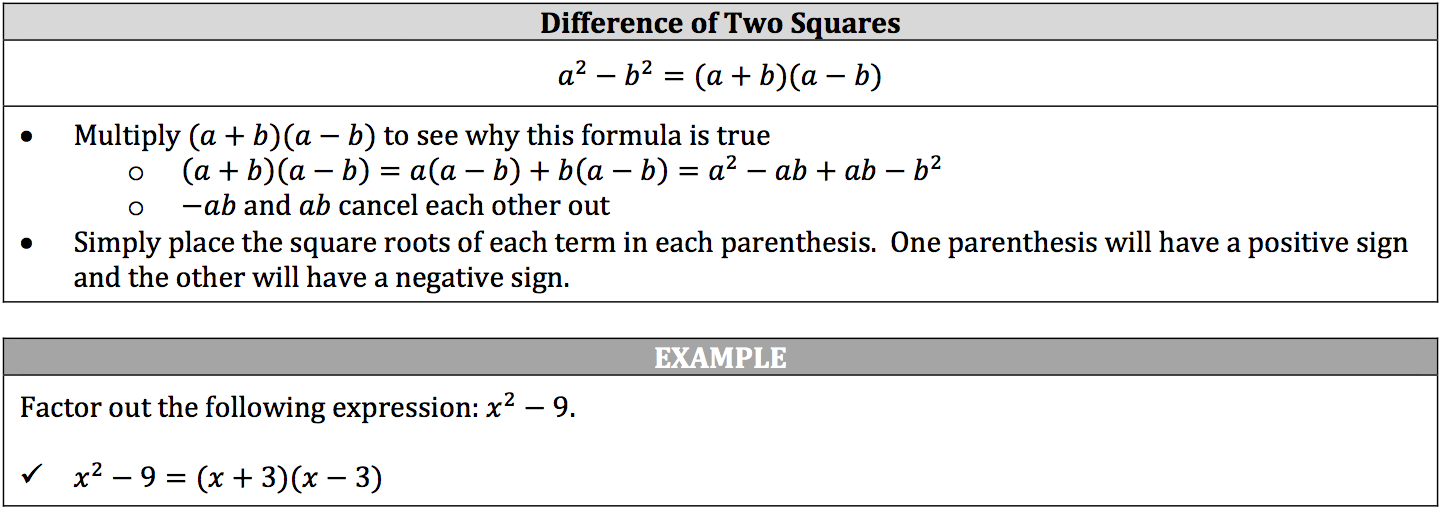Since we can multiply algebraic expressions we can factor them out. While the process of factoring is different than using a factor tree, the concept is the same. You are looking for what parts make up the whole algebraic expression. Think of factoring as the reverse of multiplying algebraic expressions.

Factoring out algebraic expressions works just like factoring out a known quantity in that nothing is being removed from the expression it is simply being broken down. If an algebraic expression cannot be factored out, then it is considered prime, just like prime numbers.

You’ll be focusing on three types of factoring for the ISEE:

• common factors
• the difference of two squares

### Using Common Factors